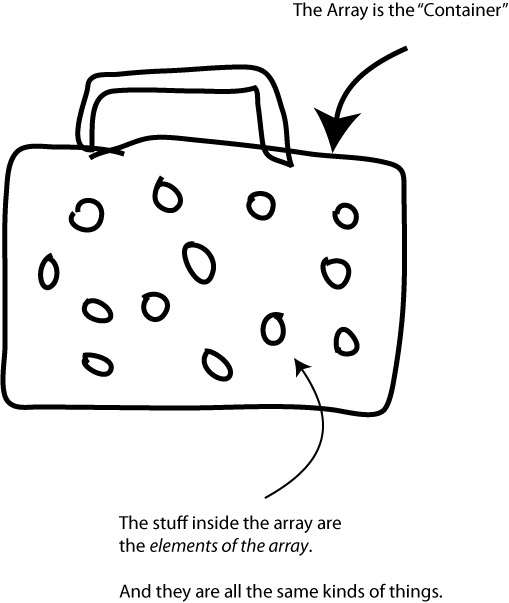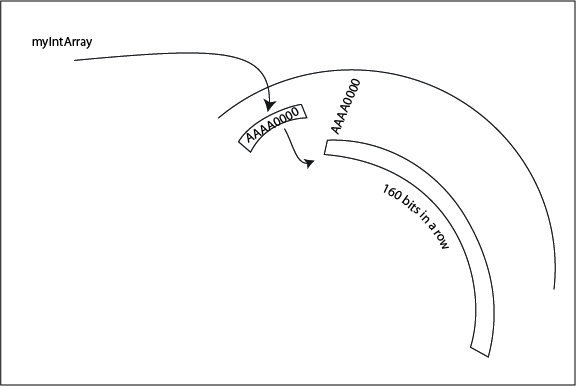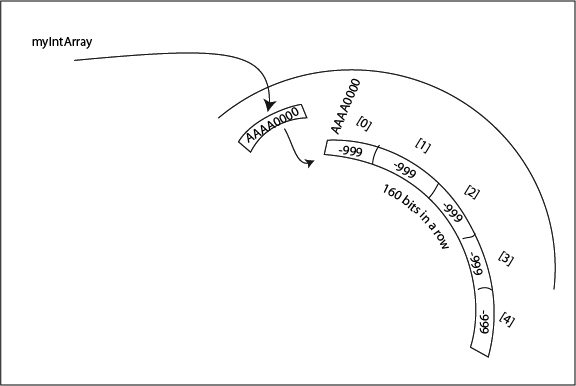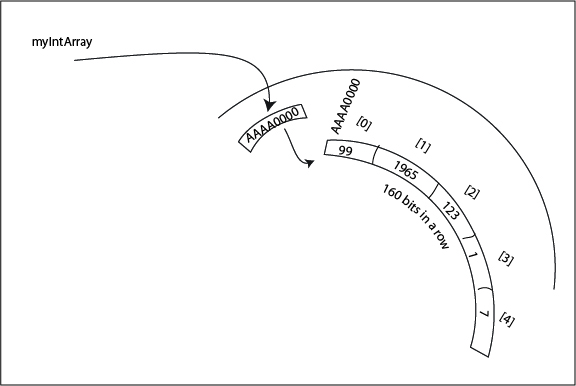Logout

--- Abstract data structures ---

5.1.4

Describe the characteristics of a two-dimensional array.

Teaching Note:

LINK One-dimensional arrays and basic algorithms.

Sample Question:

sdfsdfsf

JSR Notes:

### Important set-up and context of these notes

Since this is now HL material, with these notes, we are first going to go back and take a much more in-depth look at arrays in general. We will do that here in 5.1.4. Then, in 5.1.5 we will focus in on 2D arrays.

But in terms of this assessment statement in and of itself, the main thing in the description is as follows:

### Characteristics of 2D Arrays

The main characteristic of a 2d array is that it stores an array of arrays; i.e. each element in an array is itself a multiple item array. So in the example of a table, there are multiple rows, but each rows is itself an array (an array of column elements).

****Now back to Arrays in general, but to a deeper level than Topic 4****

### Arrays Data Types vs. Data Structures

We have alreasy looked into the nature of objects, and we have also looked at Java primitive data types, such as ints, booleans, and doubles. We briefly got introduced to Collections. Now we look at our first data structure, the array. To get straight the difference between a primitive data type, an object, and a data structure, consider the following definitions.

primitive data type - a certain basic type of data defined not only by how it is used (for example, as a character versus as a real number), but also on how much memory it occupies (a 16 bit char versus a 64 bit double). Examples: long, float, char.

object - a reference to a specific instance of a certain class made up of certain data and methods that act on that data. Examples: BufferedReader br, Scanner snr, TicketingAccount t, System.in.

data structure - a group of similar data or objects organized somehow. Examples: arrays, linked lists, binary search trees (all of which we cover in the course.)

collection - a data structure, i.e. a group of similar data or objects, which can behave in a dynamic way; it can grow or shrink in size.

As with all definitions, they will only be perfectly clear after having gained experience "on the ground" with them.

### Arrays

So, our first data structure we look at in detail is arrays.

Do note in fact, that we have seen arrays right from day 1 when we used the args array found as an argument of all main methods:

public static void main(String [] args)

You can think of an array as a container. It is a container that stores many things all of the same type. (In some programming languages you can have arrays which contain elements of various types, but not in Java.) So a simple analogy would be a carton of eggs. The carton itself is the array, the eggs are what make up the content of the carton. The individual pieces of content within the array are most properly called as "elements". So a carton of eggs is an array, with 12 egg elements. Each egg would be identified by its number, starting with 0; so egg-0, egg-1, egg-2, and so on, up to egg-11.-------------------------------------------------------------------------------------------------------------------

### Declaring Arrays

Here is how we declare an array: (We could use any type, but for the example we'll use int.)

int [] myIntArray = new int ;
(The way you shold read the above declaration is "int array myIntArray is asssigned a new int array of size five".)

What this does is finds a place in memory where there is 5 x 32 bits available (that would be a total of 160 available bits). And that block of available memory would be referred to as "myIntArray" until the array was garbage collected.

Note that the way particular elements are able to be found is due to the fact that all of the elements of the array are next to each other. The term we use to describe this is "contiguous". We say that the elements of an array are all located next to each other in a contiguous part of RAM memory big enough to accomodate them.-------------------------------------------------------------------------------------------------------------------

### Initializing Arrays

***It is very important to realize that upon declaration of the array the above way, only default values have been assigned to each element of the array; in the case of ints, they are initialized to 0. And though this isn't such a big deal, later on in the course when we are making arrays of our own objects, this will be a big deal. So it's a good habit to get into to initialize all the elements ourselves, immediately after declaring an array. So:

int [] myIntArray = new int;
myIntArray = -999;
myIntArray = -999;
myIntArray = -999;
myIntArray = -999;
myIntArray = -999;So, above, the 0th element of the array is assigned the value -999, and the "1st" element of the array is assigned -999, and so on. Do note that with 0 numbering, the elements are numbered 0 to 11, and the length, done with traditional numbering, is 12. This can cause confusion and errors, so try to remember this from the start.

(The one thing that you may wonder is if there is a way to not have to copy and paste so much; it's not too bad if there are only 12 elements, but what if there were 12,000,000. And, yes, there is a way, with looping structures that we will look at later on.)

Then, as a program actually uses the array, the values will take on more meaningful, non-default, values. Let's say, for example, this was from a program that kept a person's five favorite integers. (How 'bout that for a lame example...)

System.out.println("What is your most favourite integer?)
System.out.println("What is your second most favourite integer?)
etc.-------------------------------------------------------------------------------------------------------------------

### Here's an actual full Java example

(though at this point it won't be able to be too useful)

``` 1 import java.io.*;
2
3 public class ForArraysNotes {
4     public static void main(String[] args) {
5         try{
6             int [] gradesArray = new int;
13             System.out.println("What is the grade you got in Writing?");
15             System.out.println("What is the grade you got in Arithetic?");
17
19             System.out.println("The average grade you got in \"The Three 'R's\"" + " was " + averageGrade);
20         }
21         catch(Exception e){
22             System.out.println(e.getMessage());
23         }
24     }
25 }
```

The output for this would be:

90
What is the grade you got in Writing?
80
What is the grade you got in Arithmetic?
100
The average grade you got in "The Three R's" was 90

-------------------------------------------------------------------------------------------------------------------

### How Elements of An Array Are Located

The idea is that the computer knows fundamental two things about the array when you declare it: how big it is to be, and the data type of the items it will store. When it finds enough space to accommodate this request, it will record, as the array object variable, the address of where the array will start.

So from that point on, to find any particular element of the array in memory, it simply takes the starting address, and adds on the following number of bits: the element number multiplied by the number of bits of the data type being held.

So if an integer array called intArr started at the hexadecimal address AAAA0000, the second element of it (i.e. intArr) would start exactly 64 bits (2 x 32) on from AAAA0000, which in hexadecimal is address AAAA0040. (By the way, that's becasue the second numeric place in hexadecimal is the 16 ^ 1 place, or the 16s, and 4 groups of 16 is 64 - more on this later on in the course.)

Expressed as a formula, we locate a particular element in an array as follows:

The array's address + (the element number * size / element)

-------------------------------------------------------------------------------------------------------------------

### Index Out of Bounds Error

One thing to remember, is to make sure to only try to access an array element that actually exists. For starters, there could never be an element named with a negative sign. So, for example, in the above program, gradesArray[-2] does not exist. But neither does gradesArray. This is a very common error, in which you are trying to access the last element of the array, and you forget that arrays are numbered starting at 0.

Trying to access an array element that does not exist is only caught when running the program - no red squigglies will appear while you are coding. An error that is picked up only when a program is run is called a "runtime error". And this particular kind of runtime error is called "index out of bounds error".

-------------------------------------------------------------------------------------------------------------------

### Alternative Way of Declaring an Array

An array can be declared and initialized all in one line using braces, like as follows:

int[] myOtherIntArray = {2, 4, 23, 343, 8978};

You'll note that when it is done this way, there is no need to declare the number of elements, because it's already obvious.
And note that we would still access the elments of the array the same way, so, for example, myOtherIntArray is 343.

And there are actually Two Ways to name arrays the Non-Braces Way

(This is just here for a sense of completion - you don't really need to be aware of this for IB CS, but looking in other places like on the Internet, you may come across this.)

There are actually two ways to declare arrays the non-braces way; the name and the [] brackets can be switched around. Both of the following are syntactically correct:

int [ ] myIntArray = new int

and

int myIntArray [ ] = new int;

-------------------------------------------------------------------------------------------------------------------

### The Length Attribute of Arrays

All arrays have a length attribute, which can be accessed using dot notation. So from the above example, if we went:

System.out.println("The length of the array is " + myOtherIntAray.length);

we would get as output:

The length of the array is 5

***Note that the following line of code will always yeild an index out of bounds error (as discussed above), since the length attribute uses conventional numbering starting at 1, and the elements of an array are numbered using traditional computer numbering starting with 0:

System.out.printn(arr[arr.length]); // INDEX OUT OF BOUNDS ERROR.

Whereas this line would be fine:

System.out.println(arr[arr.length - 1]);